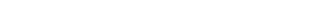## # 1.链表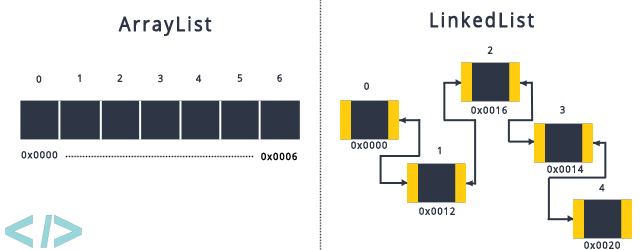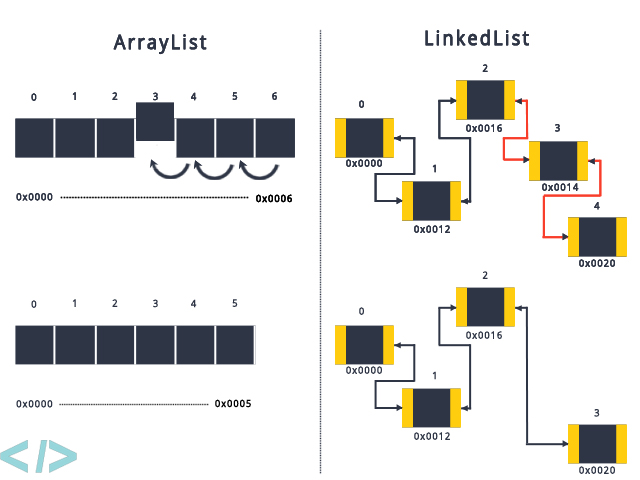### # 1.1 链表的特点

• 灵活的内存分配，不需要连续的内存空间。
• 查询慢。不能随机访问，需要迭代整个链表。时间复杂度为 O(n)，n 为链表长度。
• 修改删除快。只需要操作对应节点的数据就可以。时间复杂度为 O(1)。

### # 1.2 链表的类型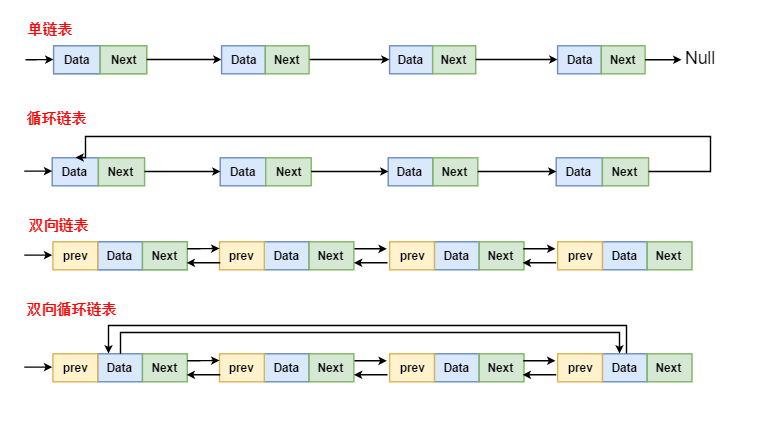### # 1.3 时间复杂对比对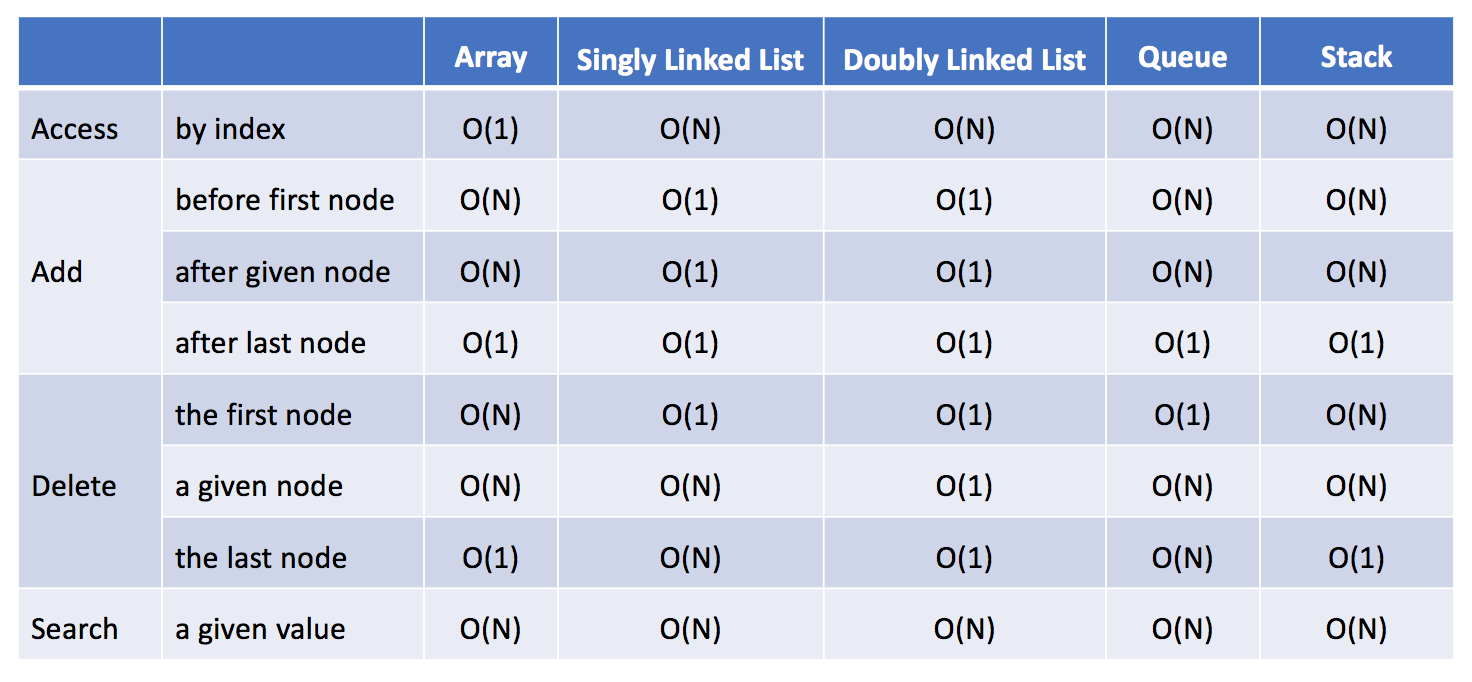## # 2.单链表

``````// 单链表-节点定义.
public class SinglyListNode {
int val;
SinglyListNode next; // 后驱节点
SinglyListNode(int x) { val = x; }
}
``````

## # 4.双向链表

``````// 双向链表-节点定义.
class DoublyListNode {
int val;
DoublyListNode next, prev; // 后驱、前驱节点
DoublyListNode(int x) {val = x;}
}
``````

``````// 寻找 index 所在的节点
private Node findCurrNode(int index) {
if (index == 0) return head;
if (index == size - 1) return tail;

Node pred;
if (index > size >> 1) { // 判断头部、尾部遍历
pred = tail;
for (int i = size - 1; i > index; i--) pred = pred.first;
} else {
for (int i = 0; i < index; ++i) pred = pred.last;
}
return pred;
}
``````

## # 参考